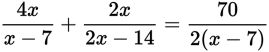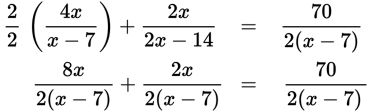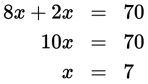# SAT Math Multiple Choice Question 253: Answer and Explanation

### Test Information

Question: 253

13.What value(s) of x satisfy the equation above?

• A. 0
• B. 7
• C. No solution
• D. Any value such that x ≠ 7

Explanation:

C

Difficulty: Hard

Category: Passport to Advanced Math / Exponents

Strategic Advice: When solving a rational equation, start by getting a common denominator. Then, you can set the numerators equal and solve for the variable. Don't forget, however: If the answer produces zero in any denominator, then it is not a valid answer.

Getting to the Answer: The denominators are almost the same already; you just need to multiply the top and bottom of the first term by 2, factor the denominator of the second term, and you'll be ready to solve the equation.Now that the denominators are all the same, you can solve the equation represented by the numerators.Be careful-this isn't the correct answer. Because there are variables in the denominator, you must check the solution to make sure it isn't extraneous, or in other words, doesn't cause a 0 in the denominator of any term. Unfortunately, if x = 7, then all of the denominators are zero (and division by zero is not possible), so the equation has no solution.